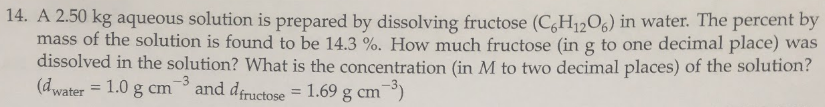# 14. A 2.50 kg aqueous solution is prepared by dissolving fructose (C6H12O6) in water. The percent by mass of the solution is found to be 14.3%. How much fructose (in g to one decimal place) was dissolved in the solution? What is the concentration (in M to two decimal places) of the solution? (dwater = 1.0 g cm^-3 and dfructose = 1.69 g cm^-3)